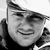# New to Qlik Sense

If you’re new to Qlik Sense, start with this Discussion Board and get up-to-speed quickly.

Announcements
cancel
Showing results for
Did you mean:Contributor II

HI,

What is the meaning of the following expression:

If(Count({\$<[Case Is Closed]={'True'} >} %CaseId)>0, Avg ([Case Duration Time]),0)+ If(Count({\$<[Case Is Closed]={'False'} >} %CaseId)>0, Avg([Case Aging]), 0)

also, what is the 0 which I colored in red

2 Solutions

Accepted SolutionsMVP

=If( Count({\$<[Case Is Closed]={'True'} >} %CaseId)>0, /*If you have any Case that is closed*/
Avg ([Case Duration Time]), /*then calc avg case duration time*/
0) /*else use zero*/
+
If( Count({\$<[Case Is Closed]={'False'} >} %CaseId)>0, /*If you have any Case that not is closed*/
Avg([Case Aging]), /*Then Calculate average Case Aging*/
0 /*Else use zero*/
)Partner - Champion III

Vegar's explanation should answer your question. For the sake of completeness, you could rewrite that expression in a pure set expression (no conditionals):

``````RangeSum(
Avg({\$<[Case Is Closed]={'True'} >} [Case Duration Time]),
Avg({\$<[Case Is Closed]={'False'} >} [Case Aging])
)``````

which may also make the intent clearer.

Logic will get you from a to b. Imagination will take you everywhere. - A Einstein
3 RepliesMVP

=If( Count({\$<[Case Is Closed]={'True'} >} %CaseId)>0, /*If you have any Case that is closed*/
Avg ([Case Duration Time]), /*then calc avg case duration time*/
0) /*else use zero*/
+
If( Count({\$<[Case Is Closed]={'False'} >} %CaseId)>0, /*If you have any Case that not is closed*/
Avg([Case Aging]), /*Then Calculate average Case Aging*/
0 /*Else use zero*/
)Partner - Champion III

Vegar's explanation should answer your question. For the sake of completeness, you could rewrite that expression in a pure set expression (no conditionals):

``````RangeSum(
Avg({\$<[Case Is Closed]={'True'} >} [Case Duration Time]),
Avg({\$<[Case Is Closed]={'False'} >} [Case Aging])
)``````

which may also make the intent clearer.

Logic will get you from a to b. Imagination will take you everywhere. - A EinsteinContributor II
Author
Thank you Vegar